International
Tables for
Crystallography
Volume B
Reciprocal space
Edited by U. Shmueli

International Tables for Crystallography (2006). Vol. B, ch. 5.1, pp. 550-551   | 1 | 2 |
https://doi.org/10.1107/97809553602060000569

## Appendix A5.1.1.

A. Authiera*

aLaboratoire de Minéralogie-Cristallographie, Université P. et M. Curie, 4 Place Jussieu, F-75252 Paris CEDEX 05, France
Correspondence e-mail: authier@lmcp.jussieu.fr

#### A5.1.1.1. Dielectric susceptibility – classical derivation

| top | pdf |

Under the influence of the incident electromagnetic radiation, the medium becomes polarized. The dielectric susceptibility, which relates this polarization to the electric field, thus characterizes the interaction of the medium and the electromagnetic wave. The classical derivation of the dielectric susceptibility, χ, which is summarized here is only valid for a very high frequency which is also far from an absorption edge. Let us consider an electromagnetic wave,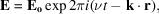incident on a bound electron. The electron behaves as if it were held by a spring with a linear restoring force and is an oscillator with a resonant frequency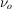. The equation of its motion is written in the following way: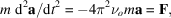where the driving force F is due to the electric field of the wave and is equal to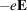. The magnetic interaction is neglected here.

The solution of the equation of motion is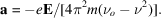The resonant frequencies of the electrons in atoms are of the order of the ultraviolet frequencies and are therefore much smaller than X-ray frequencies. They can be neglected and the expression of the amplitude of the electron reduces to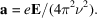The dipolar moment is therefore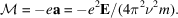von Laue assumes that the negative charge is distributed continuously all over space and that the charge of a volume element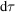is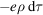, where ρ is the electronic density. The electric moment of the volume element is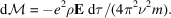The polarization is equal to the moment per unit volume: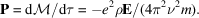It is related to the electric field and electric displacement through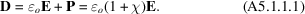We finally obtain the expression of the dielectric susceptibility,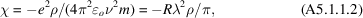where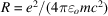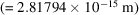is the classical radius of the electron.

#### A5.1.1.2. Maxwell's equations

| top | pdf |

The electromagnetic field is represented by two vectors, E and B, which are the electric field and the magnetic induction, respectively. To describe the interaction of the field with matter, three other vectors must be taken into account, the electrical current density, j, the electric displacement, D, and the magnetic field, H. The space and time derivatives of these vectors are related in a continuous medium by Maxwell's equations: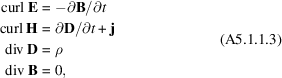where ρ is the electric charge density.

The electric field and the electric displacement on the one hand, and the magnetic field and the magnetic induction on the other hand, are related by the so-called material relations, which describe the reaction of the medium to the electromagnetic field: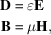whereand μ are the dielectric constant and the magnetic permeability, respectively.

These equations are complemented by the following boundary conditions at the surface between two neighbouring media: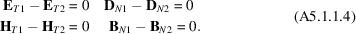From the second and the third equations of (A5.1.1.3), and using the identity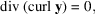it follows that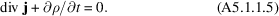#### A5.1.1.3. Propagation equation

| top | pdf |

In a vacuum, ρ and j are equal to zero, and the first two equations of (A5.1.1.3)can be written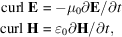where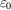andare the dielectric constant and the magnetic permeability of a vacuum, respectively.

By taking the curl of both sides of the second equation, it follows that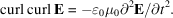Using the identity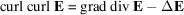, the relation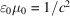, where c is the velocity of light, and noting that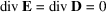, one finally obtains the equation of propagation of an electromagnetic wave in a vacuum: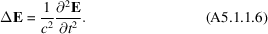Its simplest solution is a plane wave: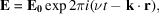of which the wavenumber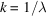and the frequency ν are related by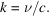The basic properties of the electromagnetic field are described, for instance, in Born & Wolf (1983).

The propagation equation of X-rays in a crystalline medium is derived following von Laue (1960). The interaction of X-rays with charged particles is inversely proportional to the mass of the particle and the interaction with the nuclei can be neglected. As a first approximation, it is also assumed that the magnetic interaction of X-rays with matter is neglected, and that the magnetic permeability μ can be taken as equal to the magnetic permeability of a vacuum,. It is further assumed that the negative and positive charges are both continuously distributed and compensate each other in such a way that there is neutrality and no current everywhere: ρ and j are equal to zero and div D is therefore also equal to zero. The electric displacement is related to the electric field by (A5.1.1.1)and the electric part of the interaction of X-rays with matter is expressed through the dielectric susceptibility χ, which is given by (A5.1.1.2). This quantity is proportional to the electron density and varies with the space coordinates. It is therefore concluded that div E is different from zero, as opposed to what happens in a vacuum. For this reason, the propagation equation of X-rays in a crystalline medium is expressed in terms of the electric displacement rather than in terms of the electric field. It is obtained by eliminating H, B and E in Maxwell's equations and taking into account the above assumptions: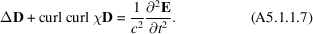Only coherent scattering is taken into account here, that is, scattering without frequency change. The solution is therefore a wave of the form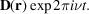By replacing D with this expression in equation (A5.1.1.7), one finally obtains the propagation equation (5.1.2.2)(Section 5.1.2.1).

In a crystalline medium, χ is a triply periodic function of the space coordinates and the solutions of this equation are given in terms of Fourier series which can be interpreted as sums of electromagnetic plane waves. Each of these waves is characterized by its wavevector,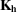, its electric displacement,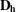, its electric field,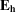, and its magnetic field,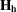. It can be shown that, as a consequence of the fact that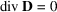and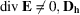is a transverse wave (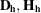andand are mutually orthogonal) whileis not. The electric displacement is therefore a more suitable vector for describing the state of the field inside the crystal than the electric field.

#### A5.1.1.4. Poynting vector

| top | pdf |

The propagation direction of the energy of an electromagnetic wave is given by that of the Poynting vector defined by (see Born & Wolf, 1983)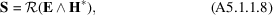where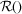means real part of ().

The intensity I of the radiation is equal to the energy crossing unit area per second in the direction normal to that area. It is given by the value of the Poynting vector averaged over a period of time long compared with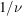: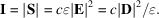### References

Born, M. & Wolf, E. (1983). Principles of optics, 6th ed. Oxford: Pergamon Press.
Laue, M. von (1960). Röntgenstrahl-Interferenzen. Frankfurt am Main: Akademische Verlagsgesellschaft.